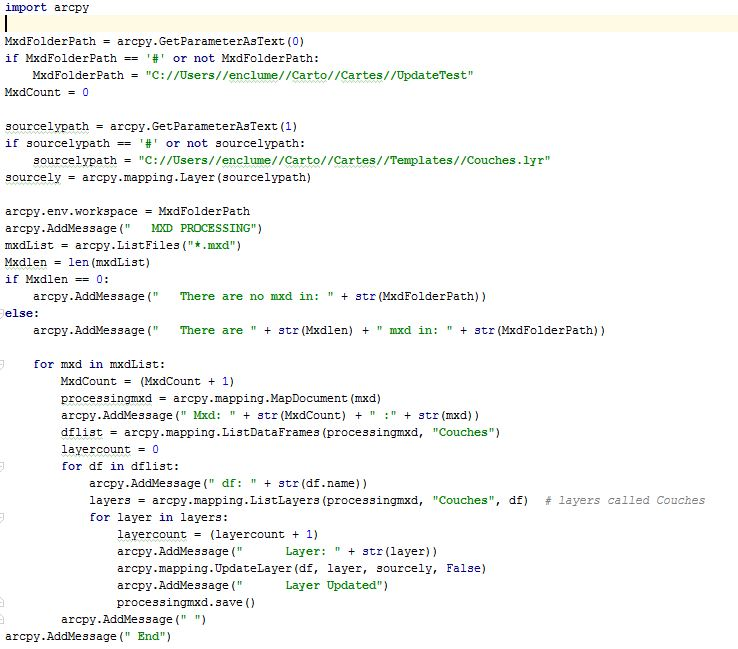# Solving my arcpy.updateLayers problem, then looping the solution...

3272
8
04-05-2016 04:46 PMNew Contributor III

Part A: I'm trying to use the code above to update layers in a mxd using a .lyr file. These layers are in a dataframe named "Couches" (there is another dataframe in the mxd). Code comes from this post.

```import arcpy.mapping as mmap

mxd = mmap.MapDocument("C:/Users/enclume/Carto/Cartes/UpdateTest/P_5_1.mxd")
DF = mmap.ListDataFrames(mxd, "Couches")
sourcely = mmap.Layer("C:/Users/enclume/Carto/Cartes/Templates/Couches.lyr")
ly = mmap.ListLayers(mxd)
mmap.UpdateLayer(DF, ly, sourcely, False)```

When I run the code everything seems fine, no error message. But when I open the mxd, nothing has changed. What am I doing wrong?

Part B: Once the problem is solved, I would like to loop this code so that it would update all mxd's in a file. How would you proceed to loop it? I saw some stuff here, but I'm far from really understanding how to apply this example to my situation. The person who wrote the code linked above mentioned the glob.glob function to loop it, but I can't find anything about it. Does anyone know how to use it?

If my mxd's folder ("C:/Users/enclume/Carto/Cartes/UpdateTest") contains some subfolders, will the looping function find all the mxd's in the folder and subfolders?

I'm new to arcpy so please be indulgent and precise...

Tags (3)
1 Solution

Accepted SolutionsNew Contributor III

Working! Now, I have to figure out how to insert a folder crawl. Thanks everyone!

```import arcpy
import os

MxdFolderPath = arcpy.GetParameterAsText(0)
if MxdFolderPath == '#' or not MxdFolderPath:
MxdFolderPath = "C://Users//enclume//Carto//Cartes//UpdateTest"
MxdCount = 0

sourcelypath = arcpy.GetParameterAsText(1)
if sourcelypath == '#' or not sourcelypath:
sourcelypath = "C://Users//enclume//Carto//Cartes//Templates//Couches.lyr"
sourcely = arcpy.mapping.Layer(sourcelypath)

arcpy.env.workspace = MxdFolderPath
mxdList = arcpy.ListFiles("*.mxd")
Mxdlen = len(mxdList)
if Mxdlen == 0:
arcpy.AddMessage("   There are no mxd in: " + str(MxdFolderPath))
else:
arcpy.AddMessage("   There are " + str(Mxdlen) + " mxd in: " + str(MxdFolderPath))

for mxd in mxdList:
MxdCount = (MxdCount + 1)
MxdPPath = os.path.join(MxdFolderPath, mxd)
processingmxd = arcpy.mapping.MapDocument(MxdPPath)
arcpy.AddMessage(" Mxd: " + str(MxdCount) + " :" + str(mxd))
dflist = arcpy.mapping.ListDataFrames(processingmxd, "Couches")
for df in dflist:
layers = arcpy.mapping.ListLayers(processingmxd, "Couches", df)  # layers called Couches
for layer in layers:
arcpy.mapping.UpdateLayer(df, layer, sourcely, False)
processingmxd.save()
8 RepliesbyMVP Esteemed Contributor

well according to this UpdateLayer—Help | ArcGIS for Desktop

it looks like the changes won't persist unless the mxd is updated... check the code examples in that linkNew Contributor III

I added "mxd.save()" in the end. Solved the first problem, thanks!byMVP Esteemed Contributor

good! ...marking the answer that solved the question, as correct, would allow others find the solution should it arise with others.New Contributor III

On another forum, someone wrote me the following code:

```import arcpy

MxdFolderPath = arcpy.GetParameterAsText(0)
if MxdFolderPath == '#' or not MxdFolderPath:
MxdFolderPath = "C://Users//enclume//Carto//Cartes//UpdateTest"
MxdCount = 0

sourcelypath = arcpy.GetParameterAsText(1)
if sourcelypath == '#' or not sourcelypath:
sourcelypath = "C://Users//enclume//Carto//Cartes//Templates//Couches.lyr"
sourcely = arcpy.mapping.Layer(sourcelypath)

arcpy.env.workspace = MxdFolderPath
mxdList = arcpy.ListFiles("*.mxd")
Mxdlen = len(mxdList)
if Mxdlen == 0:
arcpy.AddMessage(" There are no mxd in: " + str(MxdFolderPath))
else:
arcpy.AddMessage(" There are " + str(Mxdlen) + " mxd in: " + str(MxdFolderPath))

for mxd in mxdList:
MxdCount = (MxdCount + 1)
processingmxd = arcpy.mapping.MapDocument(mxd)
arcpy.AddMessage(" Mxd: " + str(MxdCount) + " :" + str(mxd))
dflist = arcpy.mapping.ListDataFrames(processingmxd, "Couches")
layercount = 0
for df in dflist:
layers = arcpy.mapping.ListLayers(processingmxd, "Couches", df)  # layers called Couches
for layer in layers:
layercount = (layercount + 1)
arcpy.mapping.UpdateLayer(df, layer, sourcely, False)
processingmxd.save()

Seems good, but I get an error message:

Traceback (most recent call last): File "<string>", line 24, in <module> File "c:\program files (x86)\arcgis\desktop10.3\arcpy\arcpy\arcobjects\mixins.py", line 611, in init assert (os.path.isfile(mxd) or (mxd.lower() == "current")), gp.getIDMessage(89004, "Invalid MXD filename") AssertionError: Invalid MXD filename

Can anyone see where is the problem?byMVP Esteemed Contributor

For one, check the indentation throughout.  for example, line 22, the "for" is indented further than the lines folloiwing.  Python is very specific when it comes to indentation.

but also right off the bat, I don't see where you set mxd.

if running from an open map doc

`mxd = arcpy.mapping.MapDocument("CURRENT")`

edit: never mind, I see you looping thru the mxdlist.   then, just work on the indentation first.New Contributor III

The identation is a copy/paste problem. It's probably my web browser but once code is added in a comment/post, it appears all messed up. Code lines are doubled and overlapping, I can't modify it. Originally it is indented as shown here:byMVP Esteemed Contributor

hope you posted the error over there...

also it is double backslashes \\ for paths, not double foreslashes //

or use 'raw' notation r'c:\somepath\somfile'  r is for raw

the error indicates the filename is wrong.  so check your environments, paths if using the mxd from disk etc.New Contributor III

Working! Now, I have to figure out how to insert a folder crawl. Thanks everyone!

```import arcpy
import os

MxdFolderPath = arcpy.GetParameterAsText(0)
if MxdFolderPath == '#' or not MxdFolderPath:
MxdFolderPath = "C://Users//enclume//Carto//Cartes//UpdateTest"
MxdCount = 0

sourcelypath = arcpy.GetParameterAsText(1)
if sourcelypath == '#' or not sourcelypath:
sourcelypath = "C://Users//enclume//Carto//Cartes//Templates//Couches.lyr"
sourcely = arcpy.mapping.Layer(sourcelypath)

arcpy.env.workspace = MxdFolderPath
mxdList = arcpy.ListFiles("*.mxd")
Mxdlen = len(mxdList)
if Mxdlen == 0:
arcpy.AddMessage("   There are no mxd in: " + str(MxdFolderPath))
else:
arcpy.AddMessage("   There are " + str(Mxdlen) + " mxd in: " + str(MxdFolderPath))

for mxd in mxdList:
MxdCount = (MxdCount + 1)
MxdPPath = os.path.join(MxdFolderPath, mxd)
processingmxd = arcpy.mapping.MapDocument(MxdPPath)
arcpy.AddMessage(" Mxd: " + str(MxdCount) + " :" + str(mxd))
dflist = arcpy.mapping.ListDataFrames(processingmxd, "Couches")
for df in dflist: